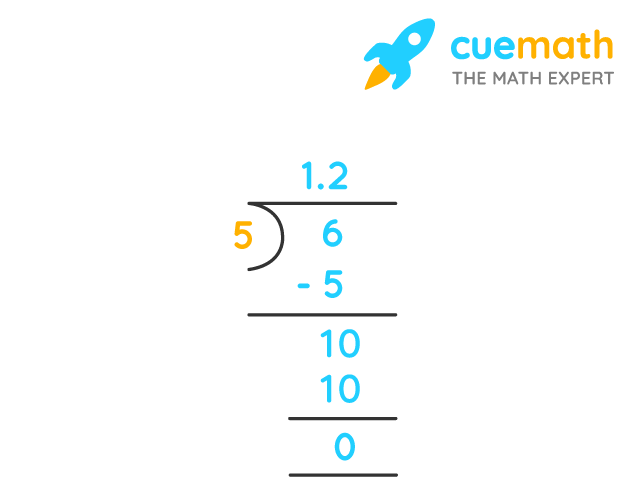# What Is 1 1/5 as a Decimal

## Question: What is 1⅕ as a decimal?

Both decimals and fractions are just two ways to represent the same number.

## Answer: 1⅕ is equivalent to 1.2 as a decimal

1⅕ is a mixed fraction. Let us find how to convert a mixed number to decimal.

## Explanation:

A mixed fraction can be represented as a decimal number in the 2-step process:

1⅕ = (1 × 5 + 1) / 5 = 6/5

6 ÷ 5 is given by:Hence, 1⅕ = 6/5 = 1.2 in decimal format.